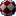# pouët.net

## Plane deformation effect

category: code [glöplog]
What is the exact formula for this effect? Don't care lighting, only plane mapping.
sure it's done more complex than just a sphere on a plane with some bumpmapping? though the sphere does look quite smooth on the edges, so perhaps you're right.
Looks like a raytraced sphere to me (x^2 + y^2 + z^2 = r^2). I guess you could get area where the ray doesn't hit look like that if you do something funky with the discriminant.
looks like a fixed deformation map scrolled around including bump mapping.

once you have the fixed (sphere) map you can also map on to the sphere an also pre calculated bump map, then use it to look up lighting.

so its a 2-3 fold table lookup effect.

atleast thats how I would make it on c64 with big pixels (80x50)
Exactly what both Preacher and Oswald said. Combined.

Do that fixed deformation map raytracing a sphere and not caring if the ray doesn't hit the surface. Just calculate the spherical inverse mapping for all the points on the screen.

In Haupex, the table is bigger than the screen so it can be scrolled. Adding bumpmap is trivial when you get to this point.
Well, I don't care about bumpmapping, just plane deformation. Could there be a formula for generating such specific map without going into 3d (during precalc)?
I think it's simply the bump-map planar-projected onto two spheres, one of which the camera is inside. Of course just pre-calculated the texture-coordinates and scrolled etc like said above.
It's a sphere mapping where I calculate z = sqrt(r^2 - x^2 - y^2) but I forget to set z to zero if z < 0. Maybe I do abs(z) later. So, it seems to wrap back in negatives z or something.
I then zoom pixels based on z iirc.Code:``` r = sqrt( x*x + y*y ); v = x*(3-sqrt(4-5*r*r))/(r*r+1); u = y*(3-sqrt(4-5*r*r))/(r*r+1); ```

http://iquilezles.org/www/articles/deform/deform.htm

implementation using processing.js (press 9 for ball)

I think the ball is overlapped onto either tunnel or another deform effect:Code:``` u = cos( a )/d; v = sin( a )/d; ```said moving tunnel effect
Oh yeah, thanks everybody. I've picked that "3-sqrt" formula and just understood something -- on chunky screens like abovementioned 80x50 (or even less) sphere mapping isn't that expressive like on 320x256 or so pixel screens. I was thinking I had wrong formulae but it turns out that the cause is different. To my taste, at least.

Btw,
Quote:

Code:``` u = cos( a )/d; v = sin( a )/d; ```

is just
Code:``` u = x/(x*x+y*y); v = -y/(x*x+y*y); ```

and is equivalent to complex F(z)=1/z mapping
Also worth considering complex square root and complex logarithm mappings (warning, they are multi-valued!).
lvd: try reducing the size and complexity of the texture.

sphere deform effect in 16x16:do it like kusma said. precalculate everything with two spheres. i think that should do the trick.
To give the impression of wrapping the texture around a sphere, the u,v distance from the center should be proportional to the distance along the surface of the sphere, which is the inverse sine of the distance on the screen, relative to the radius. Thus, to get to your u,v you should scale your x,y by

c*asin(l/r)/l where l = sqrt(x^2+y^2) and c is some texture scaling constant

This is the formula used for the bouncing ball in Peanut.
quick hack without the background:
Code:``` BYTE lookup[256*256]; float r = 4096; //radius; BYTE c = 32; //uv-multiplier //precalc for (int y = 0; y < 256; y++) for (int x = 0; x < 256; x++) { int u = x - 128; int v = y - 128; float z = sqrt(r - u*u - v*v); int a = int(u*c / z); int b = int(v*c / z); int addr = x + y * 256; lookup[addr] = a; //u-coord lookup[addr] = b; //v-coord } //loop: screen[x + y*WIDTH] = texture[(move_coord + lookup[addr] + lookup[addr] * 256) % (ISIZE)]; //un-optimized ```here's executable. kind of jerky, but whatever.
quick hack again:(without darkening).

just check z>=0 and use lookup for "tunnel" behind the sphere which is
Code:``` float d = c*sqrt(u*u + v*v); float z2 = atan2(v, u); u = cos(z2) / d; v = sin(z2) / d; lookup[addr] = 256*u; //u-coord lookup[addr] = 256*v; //v-coord```
whoa! I did something like that in my previous dos demo :)

Code:```void buildTunnel() { long x, y, i, u, v, d, m, dr, t; double r, a, b, s, z, tx, ty; i = 0; d = 160; m = 60; dr = d >> 1; t = 16; s = sqrt(dr*dr - m*m); for (y = -100; y < 100; y++) for (x = -160; x < 160; x++) { tx = x; ty = y; z = sqrt(abs(dr*dr - tx*tx - ty*ty)) + t; a = ((tx * m) / z + 0.5); b = ((ty * m) / z + 0.5); u = (a + dr); v = (b + dr); lens[i++] = (u&0xFF) + ((v&0xFF)<<8); } }```

then the usual mapping:
Code:` *((char*)scrptr++) = texture[(lens[k++]+texofs1) & 0xFFFF];`
ah, shit, lvd was the topicstarter, ffuck :)
Quote:
ah, shit, lvd was the topicstarter, ffuck :)

не переживай, он все равно не осилит:)